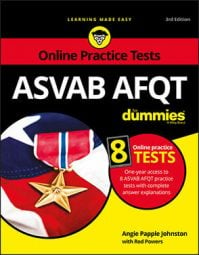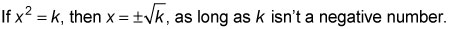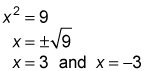##### ASVAB AFQT For DummiesSome math questions on the ASVAB AFQT may involve simple quadratic equations. These are quadratics that consist of just one squared term and a number, and they can be solved by using the square-root rule:Remember that the exponent in a quadratic is never higher than 2 (because it would then no longer be the square of an unknown but a cube or something else). An equation that includes the variable x3 or x4 is not a quadratic.

Also remember to include the plus/minus sign, which indicates that the answer is a positive or negative number. Take the following simple quadratic equation:

Solve 3x2 + 4 = 31.

1. First, isolate the variable by subtracting 4 from each side.

The result is 3x2 = 27.

2. Next, get rid of the 3 by dividing both sides of the equation by 3.

The result is x2 = 9.

3. You can now solve by using the square root rule.